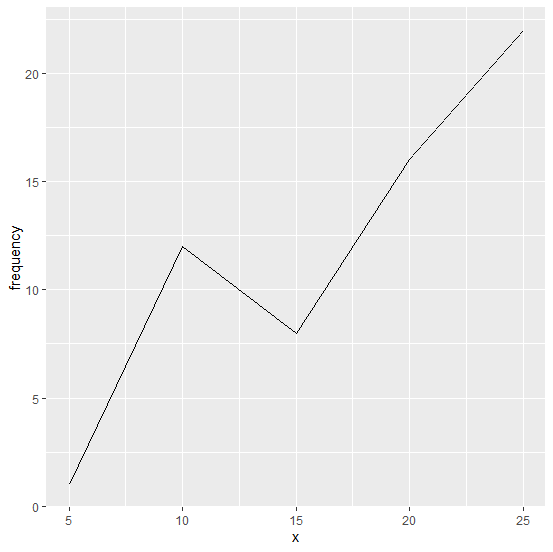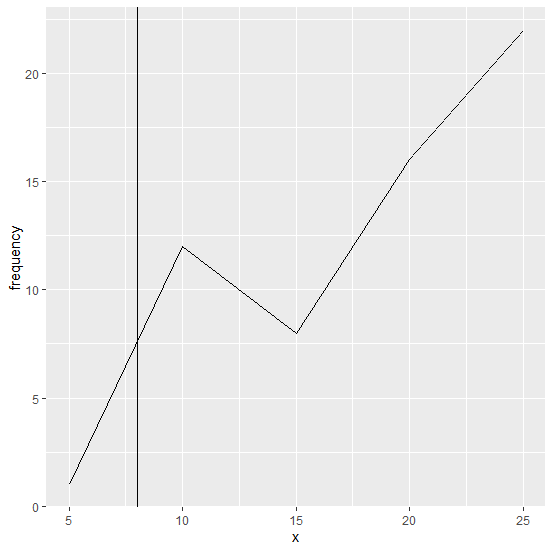# How to create a line chart using ggplot2 with a vertical line in R?

In general, the line chart is drawn to view the trend of something and we might also have some threshold point for that trend, for example, if blood pressure is plotted then we might want to show 60 mm Hg as well because this is the lowest acceptable value for blood pressure recommended by doctors. Therefore, it can be plotted as a vertical line if we want to plot blood pressures of a person. Similarly, there can be many situations where we can use a vertical line to visualize the threshold value. This can be achieved in ggplot2 with the help of geom_vline function.

## Example

Consider the below data frame −

Live Demo

set.seed(10)
x<-c(5,10,15,20,25)
frequency<-c(1,12,8,16,22)
df<-data.frame(x,frequency)
df

## Output

x frequency
1 5 1
2 10 12
3 15 8
4 20 16
5 25 22
library(ggplot2)

Creating a simple line chart −

ggplot(df,aes(x,frequency,group=1))+geom_line()

## OutputCreating the chart with a vertical line on it −

ggplot(df,aes(x,frequency,group=1))+geom_line()+geom_vline(xintercept=8)

## Output